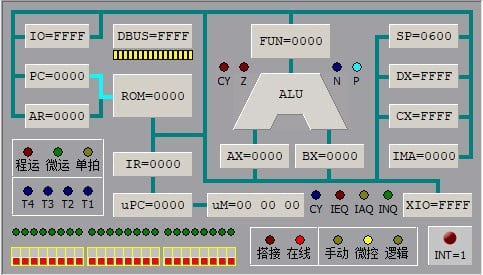## 计算机组成实践总线规则习题解答

2910 2 2018年12月7日

0 0 投票数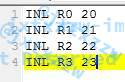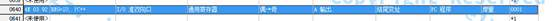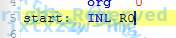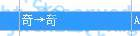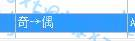W|XP|OP取值 总线规则 INL R0 INL R1 INL R2 INL R3
000 奇->奇 CX=FF12 DX=FFFF CX=12FF DX=FFFF CX=FFFF DX=FF12 CX=FFFF DX=12FF
001 奇->偶 CX=FF12 DX=FFFF CX=FF12 DX=FFFF CX=FF12 DX=FFFF CX=FF12 DX=FFFF
010 偶->奇 CX=FF34 DX=FFFF CX=34FF DX=FFFF CX=FFFF DX=FF34 CX=FFFF DX=34FF
011 偶->偶 CX=FF34 DX=FFFF CX=FF34 DX=FFFF CX=FF34 DX=FFFF CX=FF34 DX=FFFF
100 奇->奇 CX=FF12 DX=FFFF CX=12FF DX=FFFF CX=FFFF DX=FF12 CX=FFFF DX=12FF
101 奇->偶 CX=1212 DX=FFFF CX=1212 DX=FFFF CX=1212 DX=FFFF CX=1212 DX=FFFF
110 CX=FF34 DX=FFFF CX=12FF DX=FFFF CX=FFFF DX=FF34 CX=FFFF DX=12FF
111 字传递 CX=1234 DX=FFFF CX=1234 DX=FFFF CX=1234 DX=FFFF CX=1234 DX=FFFF

1、 指令系统最后2位决定的是R0~R3中的哪一个。他们的指令码不一样、散转地址一样，程序会在执行的时候自动选择是哪一个通用寄存器。

2、 当选择的是寄存器的低位时，源的奇偶字节正常工作，但是目的寄存器的奇偶字节无论怎么选择都只会存入低字节。当选择的是寄存器的高位时，源和目的的奇偶字节正常工作。

3、 如果要选择DX，OP必须为0。这也意味着很多操作在选择DX时是无法完成的，必须通过其他手段（例如分步）完成。

4、 特别注意W、XP、OP为1、1、0这组情况，是自动。即目的寄存器选的是低位，那么就自动把源的低位存放进去；目的寄存器选的是高位，那么就自动把源的高位存放进去。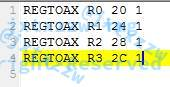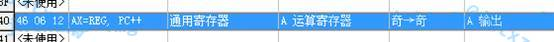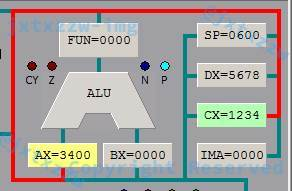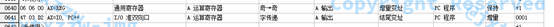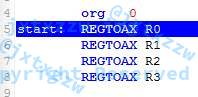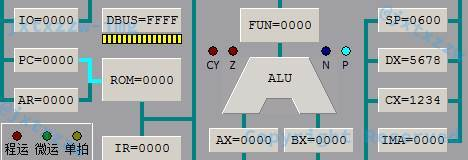W|XP|OP取值 总线规则 R0->AX R1->AX R2->AX R3->AX
000 奇->奇 3400 3400 3400 3400
001 奇->偶 0034 0034 0034 0034
010 偶->奇 3400 1200 7800 5600
011 偶->偶 0034 0012 0078 0056
100 奇->奇 3400 3400 3400 3400
101 奇->偶 3434 3434 3434 3434
110 1200 1200 5600 5600
111 字传递 1234 1212 5678 5656

1、 指令系统第5~6位决定的是R0~R3中的哪一个。他们的指令码不一样、散转地址一样，程序会在执行的时候自动选择是哪一个通用寄存器。

2、 当DX作为源寄存器的时候，XP值必须为1，否则只能选择CX。

4、 自动的总线规则，由于AX默认选择高位，所以就自动只传递高位。

5、 字传递的总线规则当选择高位时能够复制整个字、否则会复读某两位。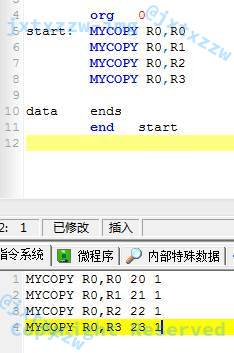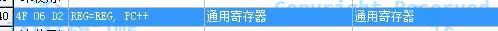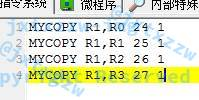RS->RD W|XP|OP取值
000 001 010 011 100 101 110 111
R0->R0 CX=1234 DX=5678 CX=1234 DX=5678 CX=1234 DX=5678 CX=1234 DX=5678 CX=1234 DX=5678 CX=3434 DX=5678 CX=1234 DX=5678 CX=1234 DX=5678
R0->R1 CX=1234 DX=5678 CX=1212 DX=5678 DX=3434 DX=5678 CX=1234 DX=5678 CX=1234 DX=5678 CX=1212 DX=5678 CX=1234 DX=5678 CX=1234 DX=5678
R0->R2 CX=1234 DX=5678 CX=1278 DX=5678 CX=1234 DX=5634 CX=1234 DX=5678 CX=1234 DX=5678 CX=7878 DX=5678 CX=1234 DX=5634 CX=1234 DX=5678
R0->R3 CX=1234 DX=5678 CX=1256 DX=5678 CX=1234 DX=3478 CX=1234 DX=5678 CX=1234 DX=5678 CX=5656 DX=5678 CX=1234 DX=1278 CX=1234 DX=5678
R1->R0 CX=1234 DX=5678 CX=3434 DX=5678 CX=1212 DX=5678 CX=1234 DX=5678 CX=1234 DX=5678 CX=3434 DX=5678 CX=1212 DX=5678 CX=1234 DX=5678
R1->R1 CX=1234 DX=5678 CX=1234 DX=5678 CX=1234 DX=5678 CX=1234 DX=5678 CX=1234 DX=5678 CX=1234 DX=5678 CX=1234 DX=5678 CX=1234 DX=5678
R1->R2 CX=1234 DX=5678 CX=7834 DX=5678 CX=1234 DX=5612 CX=1234 DX=5678 CX=1234 DX=5678 CX=7834 DX=5678 CX=1234 DX=5612 CX=1234 DX=5678
R1->R3 CX=1234 DX=5678 CX=5634 DX=5678 CX=1234 DX=1278 CX=1234 DX=5678 CX=1234 DX=5678 CX=5634 DX=5678 CX=1234 DX=1278 CX=1234 DX=5678
R2->R0 CX=1234 DX=5678 CX=1234 DX=5634 CX=1278 DX=5678 CX=1234 DX=5678 CX=1234 DX=5678 CX=1234 DX=3434 CX=1278 DX=5678 CX=1234 DX=5678
R2->R1 CX=1234 DX=5678 CX=1234 DX=5612 CX=7834 DX=5678 CX=1234 DX=5678 CX=1234 DX=5678 CX=1234 DX=1212 CX=5634 DX=5678 CX=1234 DX=5678
R2->R2 CX=1234 DX=5678 CX=1234 DX=5678 CX=1234 DX=5678 CX=1234 DX=5678 CX=1234 DX=5678 CX=1234 DX=7878 CX=1234 DX=5678 CX=1234 DX=5678
R2->R3 CX=1234 DX=5678 CX=1234 DX=5656 CX=1234 DX=7878 CX=1234 DX=5678 CX=1234 DX=5678 CX=1234 DX=5656 CX=1234 DX=5678 CX=1234 DX=5678
R3->R0 CX=1234 DX=5678 CX=1234 DX=3478 CX=1256 DX=5678 CX=1234 DX=5678 CX=1234 DX=5678 CX=1234 DX=3478 CX=1256 DX=5678 CX=1234 DX=5678
R3->R1 CX=1234 DX=5678 CX=1234 DX=1278 CX=5634 DX=5678 CX=1234 DX=5678 CX=1234 DX=5678 CX=1234 DX=1278 CX=5634 DX=5678 CX=1234 DX=5678
R3->R2 CX=1234 DX=5678 CX=1234 DX=7878 CX=1234 DX=5656 CX=1234 DX=5678 CX=1234 DX=5678 CX=1234 DX=7878 CX=1234 DX=5656 CX=1234 DX=5678
R3->R3 CX=1234 DX=5678 CX=1234 DX=5678 CX=1234 DX=5678 CX=1234 DX=5678 CX=1234 DX=5678 CX=1234 DX=5678 CX=1234 DX=5678 CX=1234 DX=5678

1、000、011、100、111是假的。

2、由实验1和实验2可知，DX作为源寄存器的时候要求XP为1、DX作为目标寄存器的时候要求OP为0，因此，当且仅当W、XP、OP为0、1、0或者1、1、0的时候，才有可能实现任意通用寄存器到通用寄存器的赋值，其余情况都不可能完成。

3、对于010来说，是完全符合预期的结果，用给定的高低位覆盖了目标的高低位。对于110来说，部分指令的执行结果符合预期，但是也有部分指令（例如R2->R1）的结果是不正确的。

CX和DX都有可能作为源寄存器，然而当且仅当XP为1的时候，源寄存器才会选择DX，否则无论RS是什么都只能选CX。对于本题来说，显然RD应该为CX或DX之一，因此，W、XP、OP的结果只剩下4种选择（010、011、110、111）。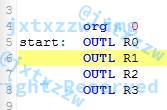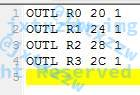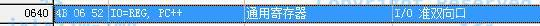W|XP|OP取值 总线规则 R0->OUT R1-> OUT R2-> OUT R3-> OUT
010 偶->奇 34FF 12FF 78FF 56FF
011 偶->偶 FF34 FF12 FF78 FF56
110 12FF 12FF 56FF 56FF
111 字传递 1234 1212 5678 5656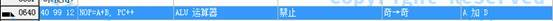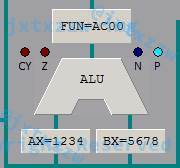W|XP|OP取值 总线规则 AX+BX
000 奇->奇 AC00
001 奇->偶 AC00
010 偶->奇 00AC
011 偶->偶 00AC
100 奇->奇 AC00
101 奇->偶 AC00
110 68AC
111 字传递 68AC

1、 总线规则的源的奇偶决定了结果存放在ALU寄存器的高字节还是低字节。

2、 当总线规则为字节的时候，无论W、XP、OP值为多少，都是AX的低字节与BX的低字节相加。

3、 当总线规则为110或者111的时候，是整个AX与整个BX相加。

0 0 投票数

2条留言（可选）如果您也有个人网站，不妨分享一下

2 评论1 (@guest_1541)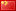Firefox 71.0 Windows 10

2019年12月26日 19:36

111IllusionBot(@_bot)Chrome 70.0.3538.110 Mac OS X 10_14_1
2018年12月7日 12:59

Warning: error_log(/home/wwwroot/www.jxtxzzw.com/wp-content/plugins/spider-analyser/#log/log-0813.txt): failed to open stream: No such file or directory in /home/wwwroot/www.jxtxzzw.com/wp-content/plugins/spider-analyser/spider.class.php on line 2900